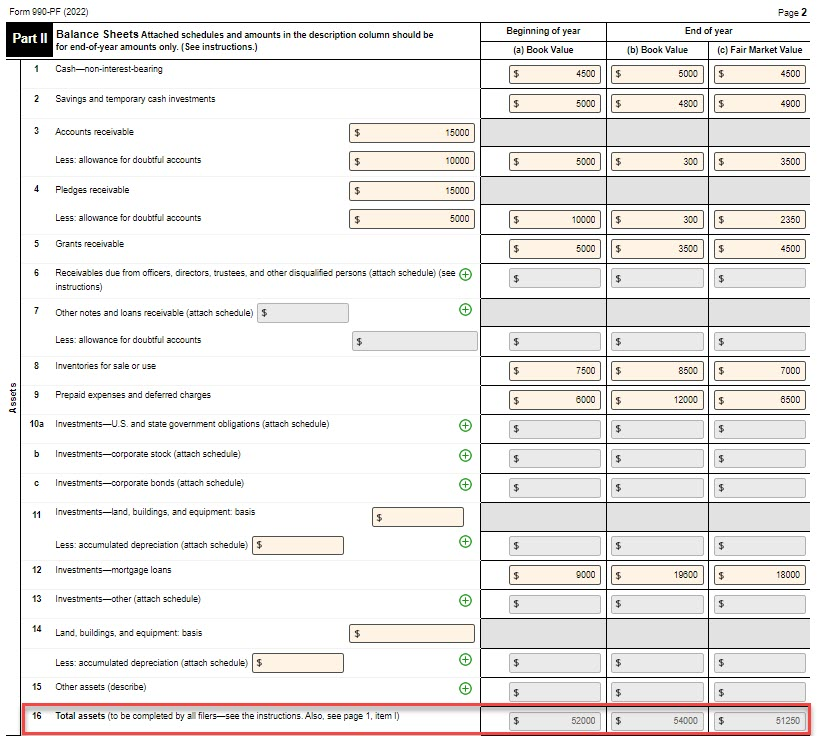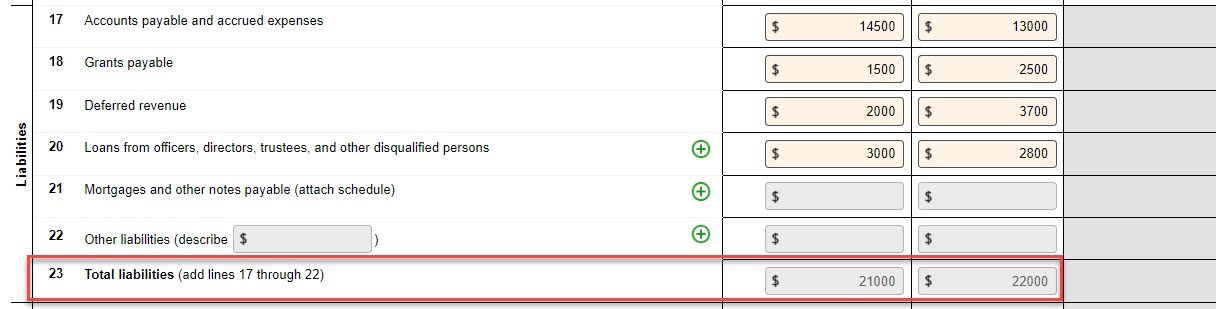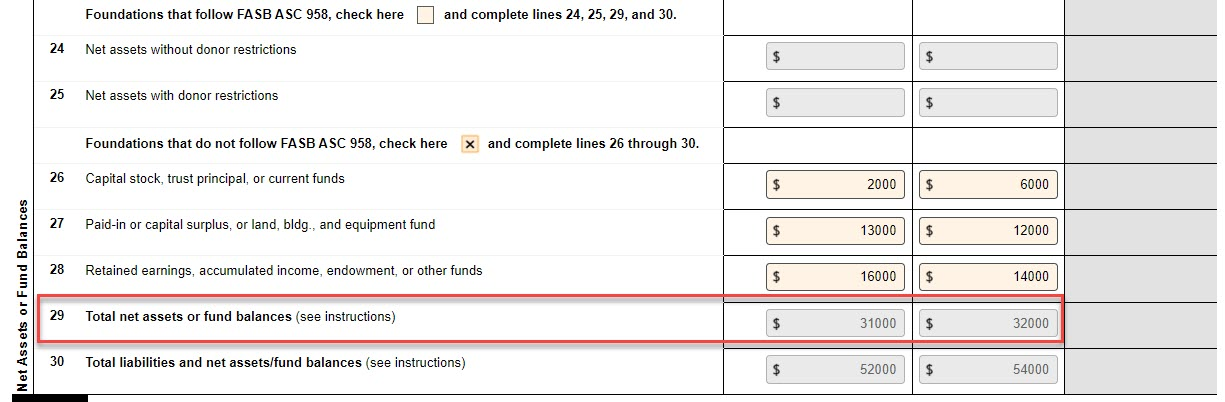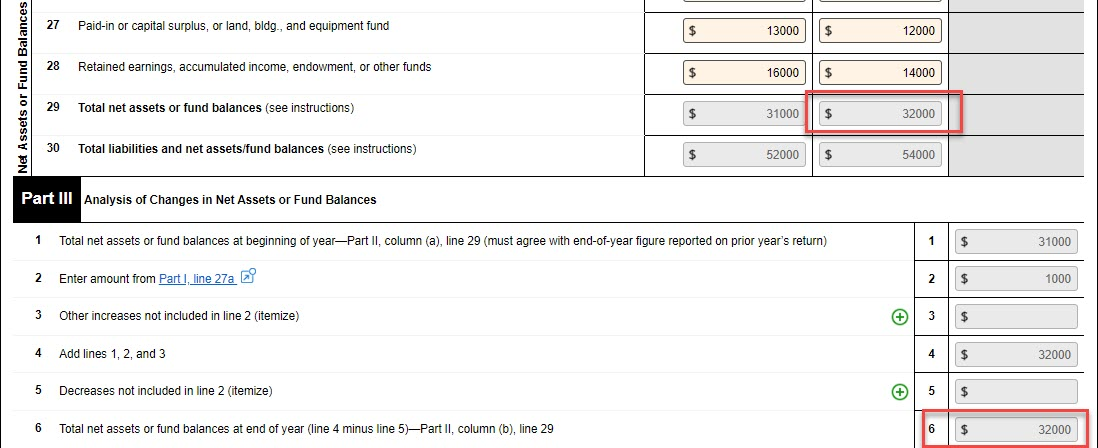# How to Complete the Balance Sheet on Form 990-PF? (Use Case) 267 views | Last modified 5/19/2023 1:04:17 AM EST |

Applicable To
990-PF 990-N 990-EZ 990 8868 1120-POL

The balance sheet on Form 990-PF provides the IRD with an overview of a private foundation's finances. The balance sheet is made up of three components.

1. Assets  - The resources owned by your foundation that have a financial value.
2. Liabilities - Debt or financial obligations of your foundation
3. Net Assets or Fund Balances - The assets left after deducting the liabilities.

The IRS requires that you provide this financial information for the beginning of the tax year (Column A), compared to the final amounts at of the end of the tax year.

The book values of the total assets reported in Section 1 must match the combined total of the total liabilities and net assets reported in Sections 2 and 3

Which means,

 Total Assets (Line 16) = Total liabilities and net assets/fund balances (Line 30)

Note: The Book value must balance out, the Fair Market Value doesn’t need to balance.

Here’s an example of a private foundation’s asset values.Calculating the foundation’s Total Assets (Line 16)

• Beginning of the tax year (A) = \$52,000 (Book Value)
• Year-end (B) = \$54,000 (Book Value)

Now, here are the foundation’s liabilities for the tax year.Calculating the foundation’s Total Liabilities (Line 23)

• Beginning of the tax year (A) = \$21,000 (Book Value)
• Year-end (B) = \$22,000 (Book Value)

This foundation follows the accounting standard FASB ASC 958, and therefore they have reported their net assets on Lines 26, 27, and 28.Calculating the foundation’s Total Net Assets or Fund Balances (Line 29)

• Beginning of the tax year (A) = \$31,000 (Book Value)
• Year-end (B) = \$32,000 (Book Value)

Now, for the last line in the Section (Line 30), you should add Lines 23 (Total Liabilities) and 29 (Total Net Assets or Fund Balances) to get the Total liabilities and Net assets/fund balances.

Total liabilities and Net assets/fund balances (Line 30) =  Line 23 + Line 29

• Beginning of the tax year (A)  = \$21,000 + \$31,000 = \$52,000
• End of the tax year (B) = \$22,000 + \$32,000 = \$54,000

Now, you can see that the values on Line 16 (A and B) are equal to Line 30 (A and B).Therefore,

 Line 16 (Total Assets) = Line 30 (Total liabilities and Net assets/fund balances)

Now, we can figure out how to balance the value of net assets in the Analysis of Changes in Net Assets or Fund Balances (Part III).

In part III, you are required to indicate your foundation’s  Total Net Assets or fund balances value at the beginning of the tax year.  (This value should be equal to the Total Net Assets or fund balances value reported last year).

Tax990 will pull this amount from Part II, Line 29, A (Total Net Assets of Fund Balances at the beginning of the tax year).

 Part III, Line 1 (Total net assets or fund balances at the year-beginning)  = Part II, Line 29 - A (Total net assets or fund balances at the year-beginning)Now, based on the revenue and expenses you entered in Part I, the amount will be calculated for Part III, Line 2. Based on the difference, the Total Net Assets or Fund Balances value at the end of the tax year will be calculated in Line 6

In the example, we determined the Total Net Assets or Fund Balance at the end of the year (Line 29, B) to be \$32,000. The value on Line 6 should be equal to this.

 Part III, Line 6 (Total net assets or fund balances at the year-end)  = Part II, Line 29 - B (Total net assets or fund balances at the year-end)As you can see in the example above, the values are balanced. Therefore, we’ve completed the balance sheet and reconciliation.